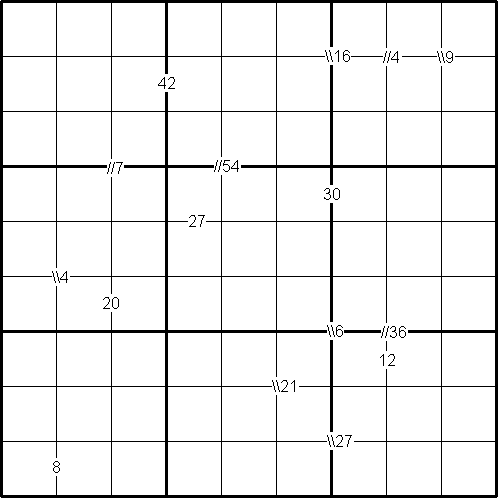#### You may also likeMake a set of numbers that use all the digits from 1 to 9, once and once only. Add them up. The result is divisible by 9. Add each of the digits in the new number. What is their sum? Now try some other possibilities for yourself!### Double Digit### More Mods

What is the units digit for the number 123^(456) ?

# Integrated Product Sudoku

##### Age 11 to 16Challenge Level

by Henry Kwok### Rules of Integrated Product Sudoku

Like the standard sudoku, this sudoku variant has two basic rules:
1. Each column, each row and each box (3$\times$3 subgrid) must have the numbers 1 to 9.
2. No column, row or box can have two squares with the same number.
The puzzle can be solved with the help of small clue-numbers which are either placed on the border lines between selected pairs of neighbouring squares of the grid or placed after slash marks on the intersections of border lines between two diagonally adjacent squares.

Each small clue-number is the product of two digits in the two squares that are horizontally or vertically or diagonally adjacent to each other. The position of each pair of diagonally adjacent squares is indicated by either two forward slash marks // or two backward slash marks \\.

For example:
• the //36 on intersection of border lines between the diagonally adjacent squares (6, 8) and (7, 7) means that possible pairs of numbers in the squares are: 4 and 9 or 9 and 4 or 6 and 6.
• the \\6 on intersection of border lines between the diagonally adjacent squares (6, 6) and (7, 7) means that possible pairs of numbers in the squares are: 1 and 6, 6 and 1; 2 and 3, or 3 and 2.
• finally, the clue-number 8 on the border line betweeen the squares (9, 1) and (9, 2) means that possible pairs of numbers for these squares can be from the following combinations: 1 and 8, 8 and 1; 2 and 4, or 4 and 2.